# Fermat-Catalan conjecture

## Statement

The equation:with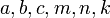positive integers such thatare relatively prime and:has only finitely many solutions, where, in the special case that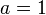or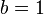, we count all solutions with distinct values of the corresponding exponent as equivalent.

(Note that sometimes the conjecture is written with strict inequality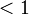. This is an equivalent formulation because in all the cases of exact equality with, it is known that there are no solutions).

## Status

The conjecture is open. A certain finite list of solutions has been found, but the conjecture does not claim that this is the complete list. Note that to avoid unnecessary duplication, we do not list the mirror solutions to each solution thatcan be obtained by interchangingwithandwith. The solutions found so far are:

Equation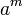Value of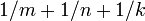Comment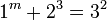,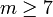1 2 3 - 3 2 1 8 9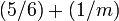Catalan's conjecture states that this is the only solution with. That conjecture has been proved.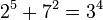2 7 3 5 2 4 32 49 81 0.95 = 19/20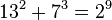13 7 2 2 3 9 169 343 512 17/18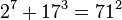2 17 71 7 3 2 128 4913 5041 41/423 11 122 5 4 2 243 14641 14884 0.95 = 19/2033 1549034 15613 8 2 3 1406408618241 2399506333156 3805914951397 23/24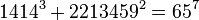1414 2213459 65 3 2 7 2827145944 4899400744681 4902227890625 41/429262 15312283 113 3 2 7 794537372728 234466010672089 235260548044817 41/42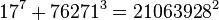17 76271 21063928 7 3 2 410338673 443688652450511 443689062789184 41/4243 96222 30042907 8 3 2 11688200277601 890888060733048 902576261010649 23/24

## Relation with other facts/conjectures

### Stronger facts and conjectures

Conjecture Statement Status Proof of implication
abc conjecture For every,there existssuch that for all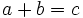,open abc conjecture implies Fermat-Catalan conjecture

### Other related conjectures and facts

Conjecture/fact Statement Status Nature of relationship
Catalan's conjecture The only solution to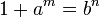for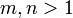andpositive is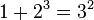proved If a complete set of solutions to the Fermat-Catalan conjecture is found and proved to be complete, Catalan's conjecture would be a special case.
Fermat's last theoremhas no solutions forandpositive integers proved The Fermat-Catalan conjecture would imply that there are only finitely many solutions. The Fermat-Catalan conjecture along with an explicit list of solutions would prove Fermat's last theorem.
Beal conjecture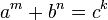has no solutions for relatively primewith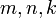all at leastThe Fermat-Catalan conjecture would imply that there are only finitely many solutions. If none of the solutions to the Fermat-Catalan problem provides a counterexample to the Beal conjecture, this would prove the Beal conjecture.
Faltings' theorem This states that certain kinds of algebraic curves have only finitely many rational points proved Faltings' theorem implies that for fixed choice of, there are only finitely many solutions. The Fermat-Catalan conjecture goes further: it says that for all but finitely many permissible choices of, there are no solutions.